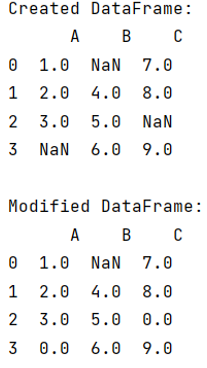# Pandas dataframe fillna() only some columns in place

Given a Pandas DataFrame, we have to use fillna() only some columns in place.
By Pranit Sharma Last updated : September 22, 2023

## pandas.DataFrame.fillna() Method

This method is used to replace the null values with a specified value which is passed as a parameter inside this method.

## Syntax

The syntax of the fillna() method is:

```DataFrame.fillna(
value=None,
method=None,
axis=None,
inplace=False,
limit=None,
downcast=None
)
```

To apply this method to specific columns, we need to define the specific columns at time of function calling.

Note

To work with pandas, we need to import pandas package first, below is the syntax:

```import pandas as pd
```

Let us understand with the help of an example,

## Program for pandas dataframe fillna() only some columns in place

```# Importing pandas package
import pandas as pd

# Importing numpy package
import numpy as np

# Creating a dictionary
d = {
'A':[1,2,3,np.NaN],
'B': [np.NaN,4,5,6],
'C':[7,8,np.NaN,9]
}

# Creating an empty DataFrame
df = pd.DataFrame(d)

# Display DataFrame
print("Created DataFrame:\n",df,"\n")

# Filling 0 in place of NaN values
df[['A','C']] = df[['A','C']].fillna(value=0)

# Display modified DataFrame
print("Modified DataFrame:\n",df)
```

### Output

The output of the above program is: